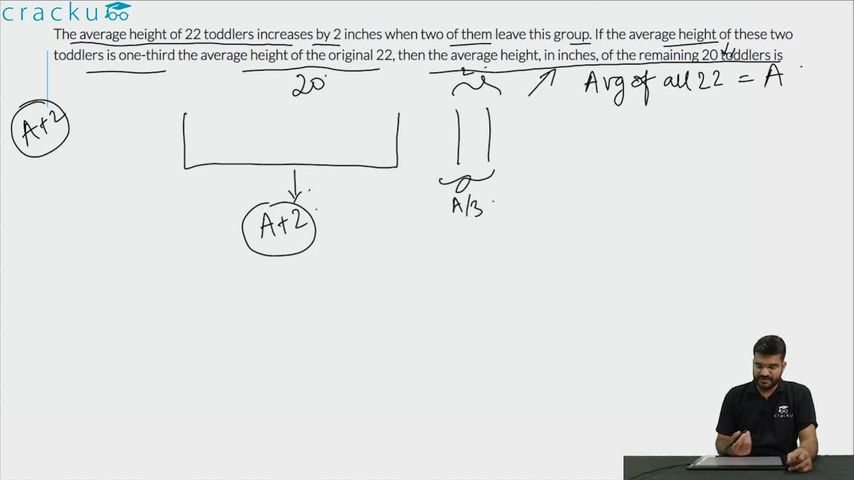Question 72

# The average height of 22 toddlers increases by 2 inches when two of them leave this group. If the average height of these two toddlers is one-third the average height of the original 22, then the average height, in inches, of the remaining 20 toddlers is

Solution

Let the average height of 22 toddlers be 3x.
Sum of the height of 22 toddlers = 66x
Hence average height of the two toddlers who left the group = x
Sum of the height of the remaining 20 toddlers = 66x - 2x = 64x
Average height of the remaining 20 toddlers = 64x/20 = 3.2x
Difference = 0.2x = 2 inches => x = 10 inches
Hence average height of the remaining 20 toddlers = 3.2x = 32 inches

### View Video Solution